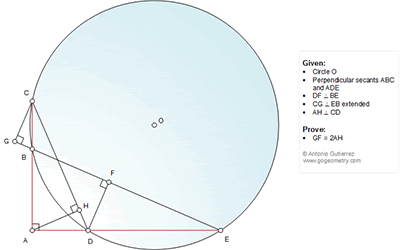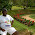## Wednesday, October 7, 2015

### Geometry Problem 1150: Circle, Perpendicular Secants, 90 Degrees, Congruence

Geometry Problem. Post your solution in the comments box below.
Level: Mathematics Education, High School, Honors Geometry, College.

Click the diagram below for more details.#### 7 comments:

1.Extend AH to M which is on BE. < MEA = < ACD = < EAM = < GCA = < DCM since Tr. ACD & MCD are therefore congruent. So 2AH = AM = ME = BM = AG

Now I need to show that BM = GF or that GB = FM which step eludes me still. Dear Antonio could u help?

Sumith Peiris
Moratuwa
Sri Lanka

1.Am I on the right track Antonio?

2.Dear Sumith,
In your first statement < MEA = < ACD = < EAM = < GCA = < DCM
why <DCM = < GCA = ....

Thanks
Antonio

2.Extend CG and EA let be P point where them meet each other. Draw MN //GF9Through A). Triangles PMA kongruent to AHD(PCD isoceles) PMA kongruent to ADN => MA=AN=AH

1.3.CBDE is cyclic, so <BEA=<ACD (1), CGAE is cyclic, so <BEA=<BCG (2), hence BC is bisector of <GCD (3). Let M be the intersection of AD and CG, N the intersection of BC and DF; from (3) and AD_|_BC, CMND is a rhombus. GF is one of its altitudes, and AH is half of another altitude, done.

4.Because CBDE is cyclic, a=<BED=<BCD
Because CGAE is cyclic, <BED=<GCB=a
Therefore, <GCD=2a.
GF=CD*sin(2a)=2CDcos(a)sin(a)=2cos(a)(CDsin(a))=2cos(a)AD.
Note that from similar triangles, <HAD=<ACD=a, so ADcos(a)=AH.
Plugging this into previous equation gives GF=2cos(a)AD=2AH.